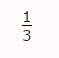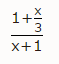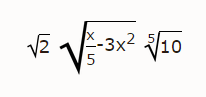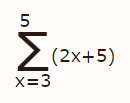Games
Problems
Go Pro!

# Formatting Equations for Website Display

The Equation Formatter is one of the powerful tools you have access to as a Pro Member at The Problem Site.  This feature is available to you as you create content in either the Problems or Reference sections of the site.

In the following sections you will see examples of how this feature can be used in developing your content.  These examples will range from simple to complex, and cover all the commands available for formatting.  Please note that we are always considering new features to add, so if you are a Pro Member, please drop us a note if you have a feature request.

## Exponents

Exponents are the simplest items to insert in your content; either use the superscript button in the editor toolbar, or use the carat character.  If you are using the carat key, and you have multiple characters to turn into an exponent, be sure to put parentheses around them.  For example, x^(2n+1) will display with 2n+1 superscripted (note that the parentheses will not appear on the page; they are there purely to tell the equation editor how to display the content).  If you leave out the parentheses, only the number two will be superscripted.

## Fractions

Including fractions is as simple as putting the text FR{1,3} in your content.  The site will parse this as "a fraction with numerator 1 and denominator 3."  It will appear in your content as shown below.More complex fractions can be created by inserting a fraction within a fraction, as this example shows:

FR{1+FR{x,3},x+1}, which displays as shown below.To include radicals (square roots, cube roots, etc.) use the SQR{} tag or the RAD{} tag.  Use the SQR tag if you are displaying a square root, or the RAD tag if you are displaying a higher-order root, such as a cube root, nth root, etc.  The RAD tag has two parameters.  The first parameter is the order of the root, and the second is the number or expression which will appear under the radical.

Here are some examples, SQR{2}, SQR{FR{x,5}-3x^2}, RAD{5,10}.  Note in the image below that the radical symbol automatically resizes to fit the expression inside.  This allows you to create both simple and complex radical expressions.## Summations (Sigma Notation)

Use the SUM{} tag to create sigma notation.  The SUM tag takes three parameters.  The first parameter is the starting variable value, the second is the ending variable value, and the third is the expression to be evaluated.  For example, SUM{x=3,5,(2x+5)} will appear like this:Like us on Facebook to get updates about new resources